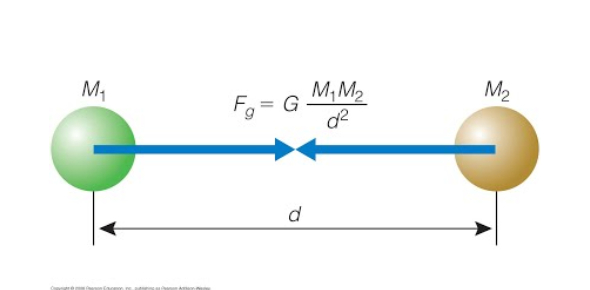# Newton's Three Laws And Gravitation: Quiz!

10 Questions | Total Attempts: 146SettingsWhat do you know about Newton’s three laws and gravitation? Do you think you can pass this quiz? In this quiz, you will encounter questions like which one of Newton’s laws describes the relationship between mass, acceleration, and force, which one of Newton’s laws deals with inertia, what are unbalanced forces, and when does friction occur. This valuable quiz will tell you all about Newton’s three laws of gravity. Go get ‘em.

• 1.
Which one of Newtons Laws states "For every action there is an equal and opposite reaction"?
• A.

First Law

• B.

Second Law

• C.

Third Law

• D.

Law of Gravitation

• 2.
Which one of Newton's Laws describes the relationship between Mass, Acceleration, and Force?
• A.

First Law

• B.

Second Law

• C.

Third Law

• D.

Law of Gravitation

• 3.
Which one of Newton's Laws deals with Inertia?
• A.

First Law

• B.

Second Law

• C.

Third Law

• D.

Law of Gravitation

• 4.
The Law of Gravitation states:
• A.

For every action there is an equal and opposite reaction

• B.

Force is the product of Mass and Acceleration

• C.

Every object pulls on every other object

• D.

Objects in motion stay in motion unless acted upon by an unbalanced force.

• 5.
Inertia is:
• A.

The tendency of an object to resist changes in motion

• B.

The amount of matter in an object

• C.

The distance an object will travel if affected by an unbalanced force

• D.

The result of adding all of the forces acting on an object

• 6.
Unbalanced forces are:
• A.

A non-zero net force.

• B.

What happens when all forces acting on an object cancel each other out and the object does not move.

• C.

The force that resists motion.

• D.

The tendency of an object to resist changes in motion

• 7.
Friction happens when:
• A.

Two objects slide across each other

• B.

An object falls through the air

• C.

Wheels on a car roll on the pavement

• D.

All of the above

• 8.
With modern technology, friction can be totally eliminated.
• A.

True

• B.

False

• 9.
Friction can be a good thing when:
• A.

You add oil to an engine to reduce wear and tear.

• B.

• C.

You sand an object to reduce the rough spots.

• D.

You add bearings to a wheel to make it roll smoother.

• 10.
If Joe pushes a box to the left with 20n of force, and Sally pushes the box to the right with 15n of force, the box will:
• A.

Not move.

• B.

Move towards the right with 5n of force.

• C.

Move towards the left with 5n of force.

• D.

Move towards the left with 35n of force.

Related TopicsBack to top